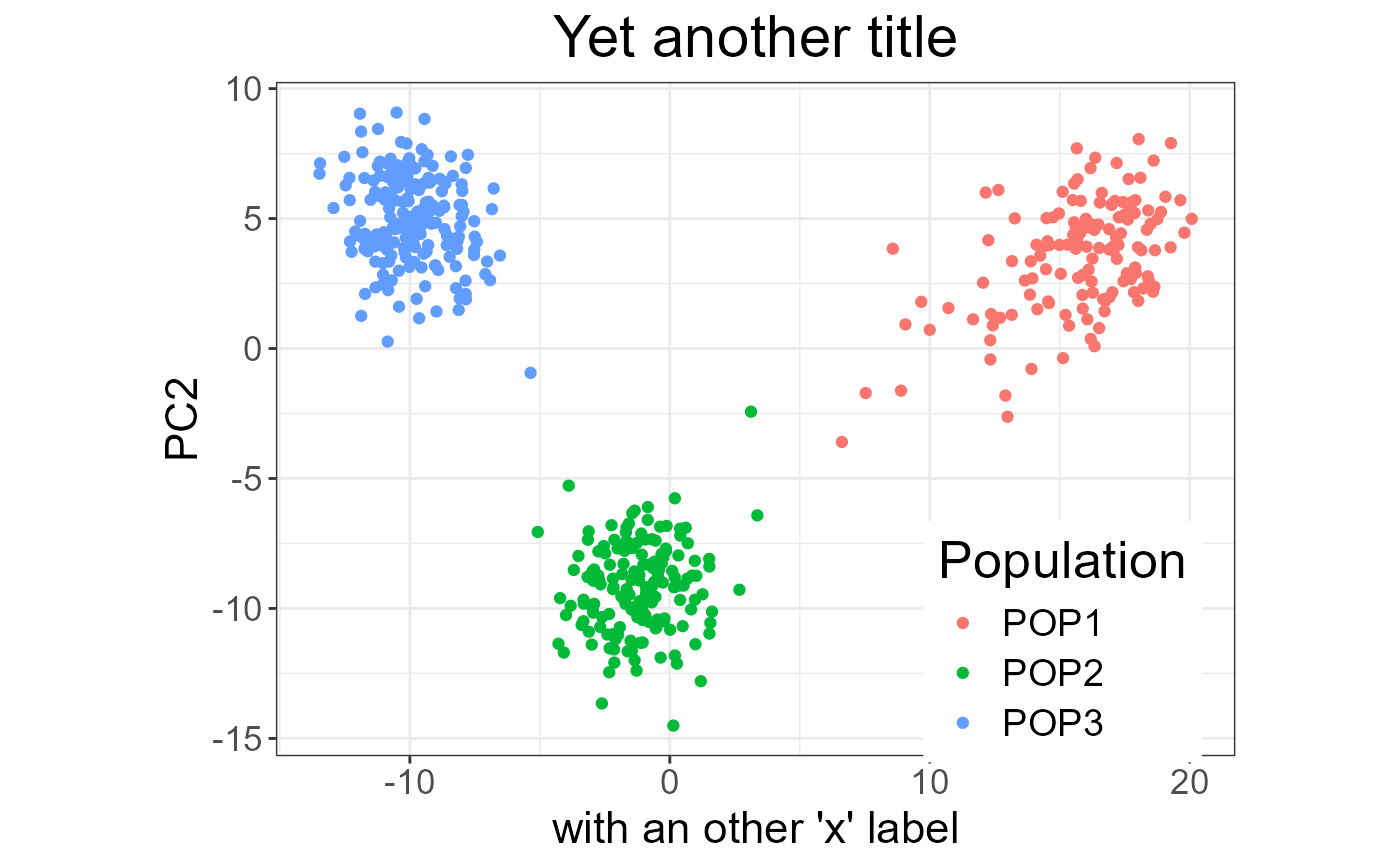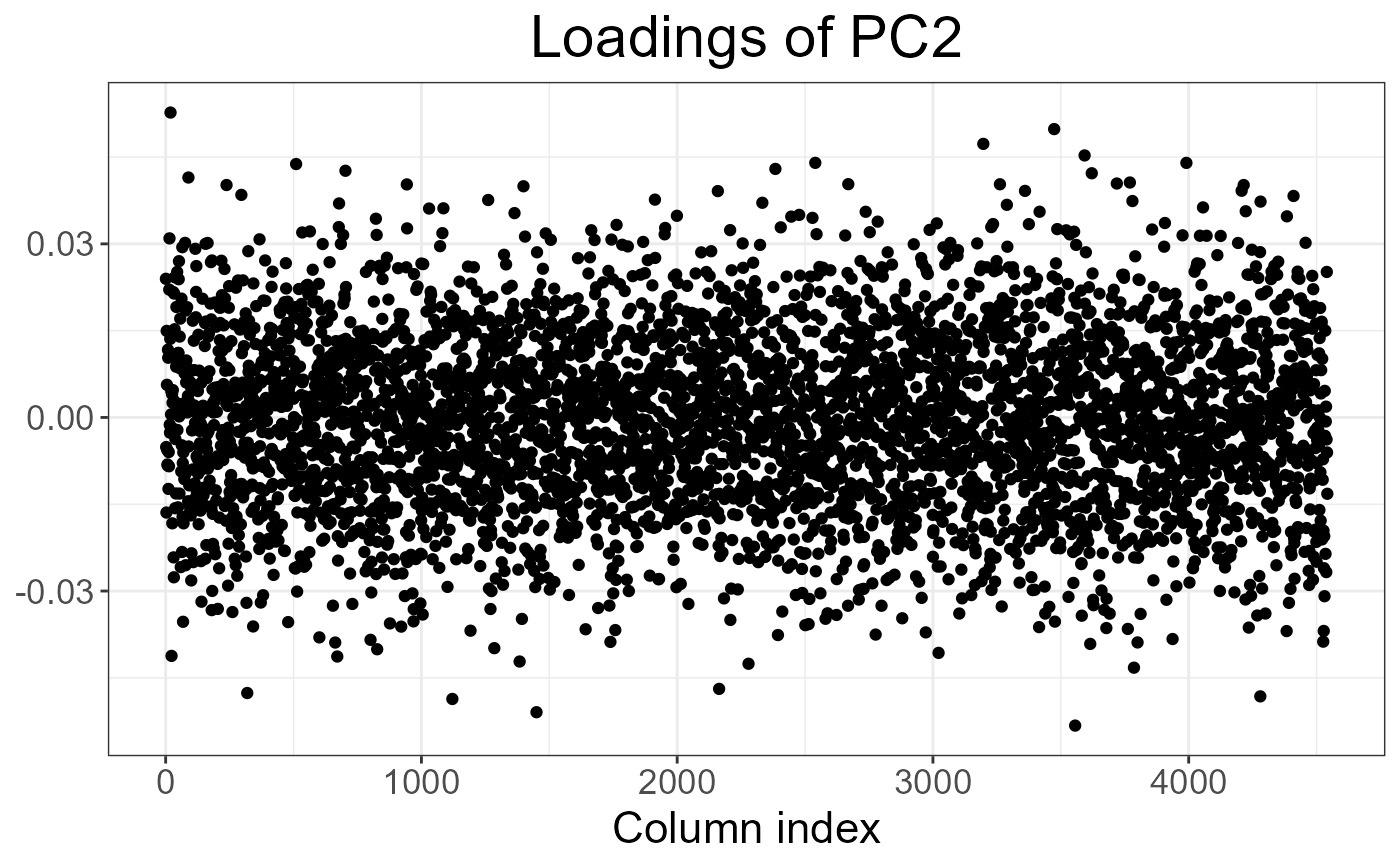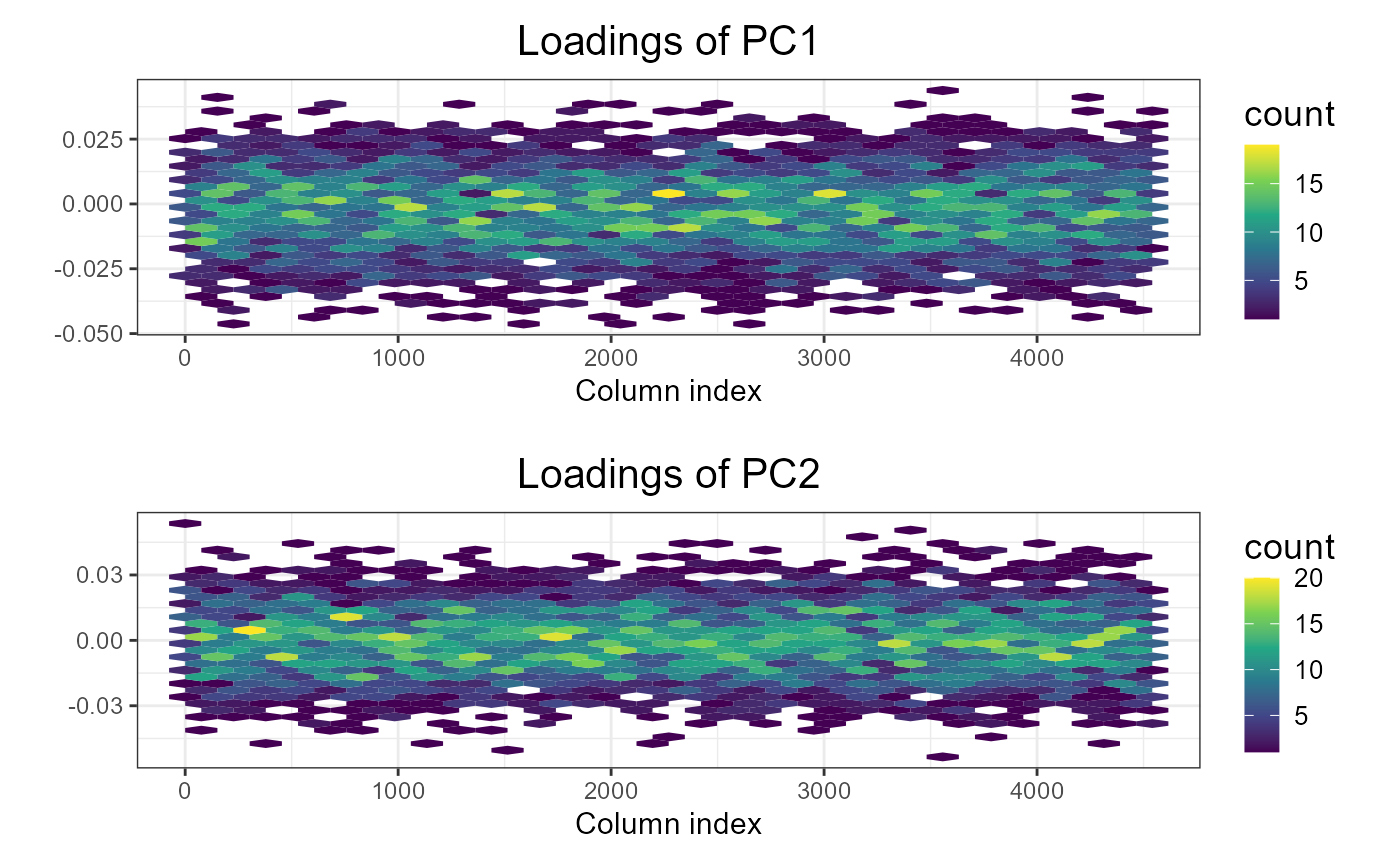Plot method for class big_SVD.

# S3 method for big_SVD
plot(
x,
nval = length(x\$d),
scores = c(1, 2),
ncol = NULL,
coeff = 1,
viridis = TRUE,
cols = 2,
...
)

Value

A ggplot2 object. You can plot it using the print method. You can modify it as you wish by adding layers. You might want to read this chapter to get more familiar with the package ggplot2.

Examples

set.seed(1) X <- big_attachExtdata() svd <- big_SVD(X, big_scale(), k = 10) # screeplots plot(svd) # 3 PCs seems "significant"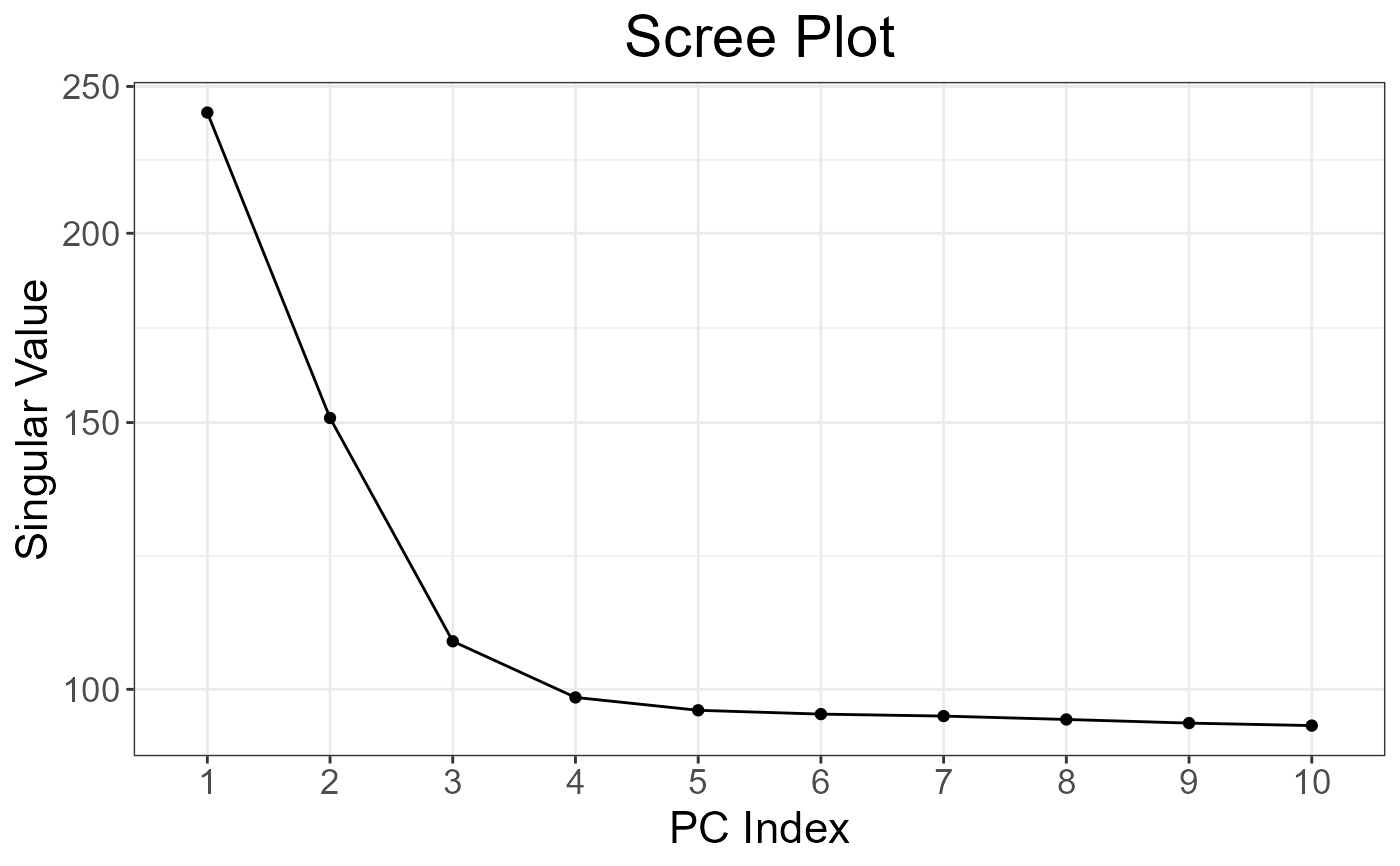plot(svd, coeff = 1.5) # larger font for papers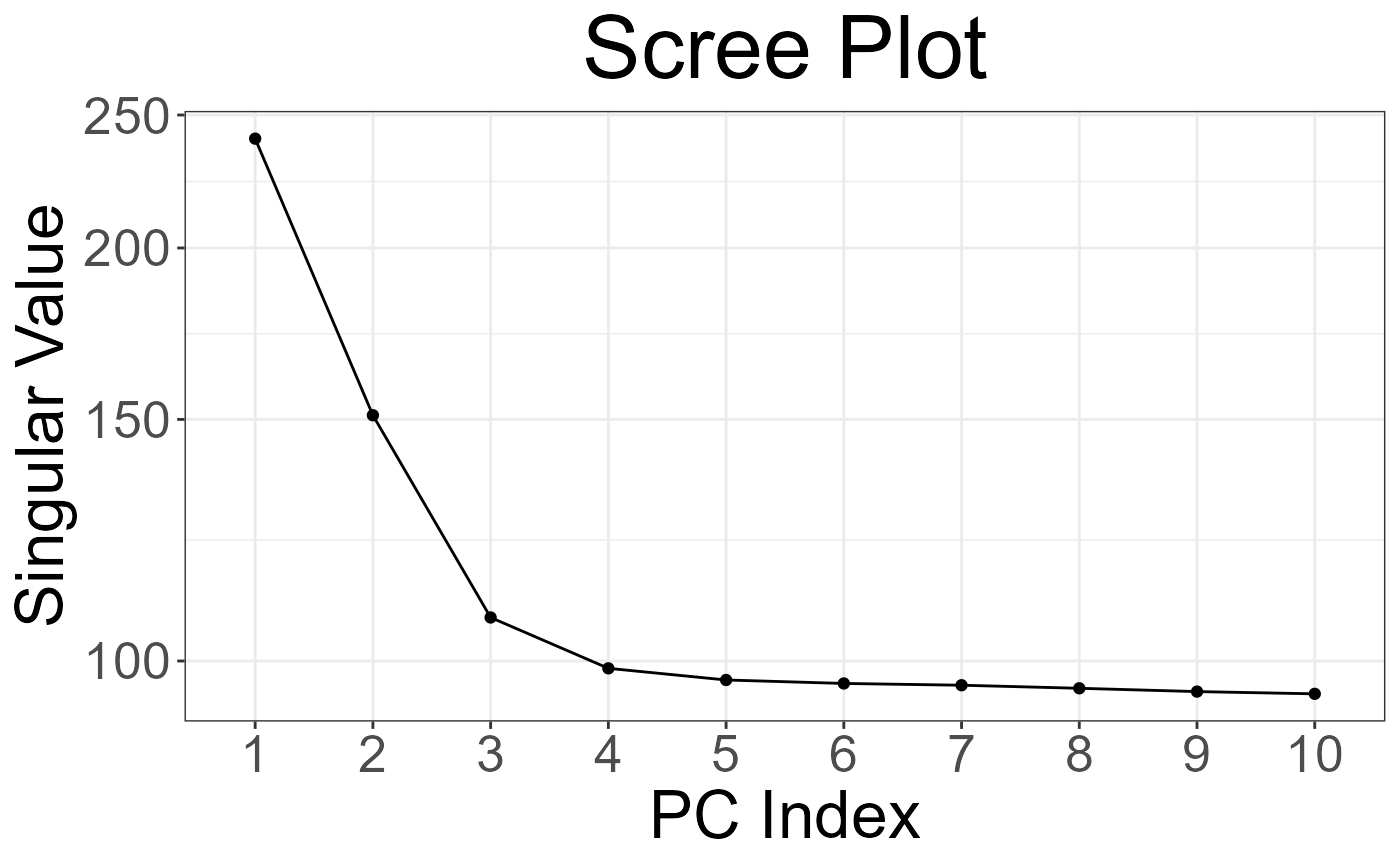# scores plot plot(svd, type = "scores") # first 2 PCs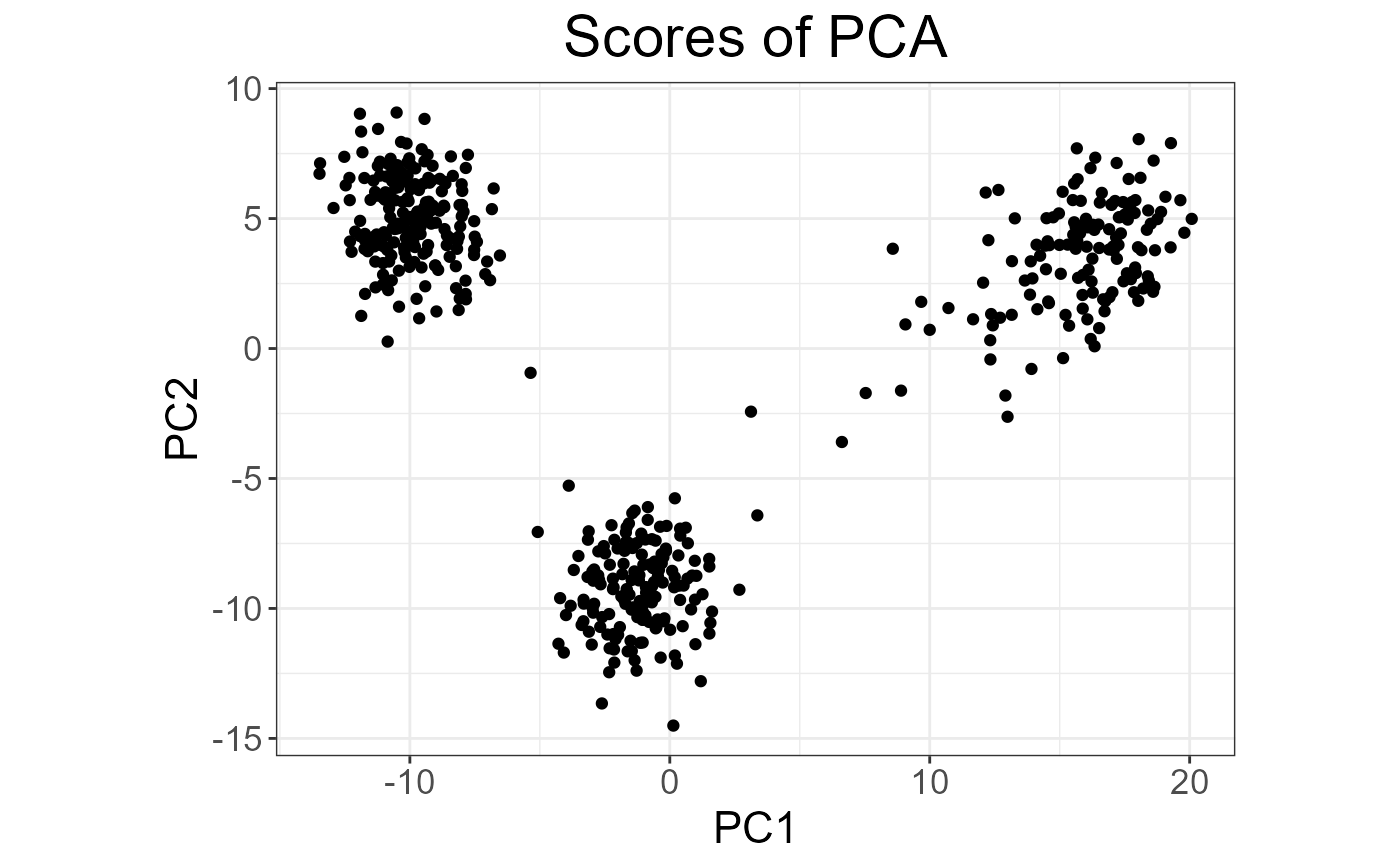plot(svd, type = "scores", scores = c(1, 3))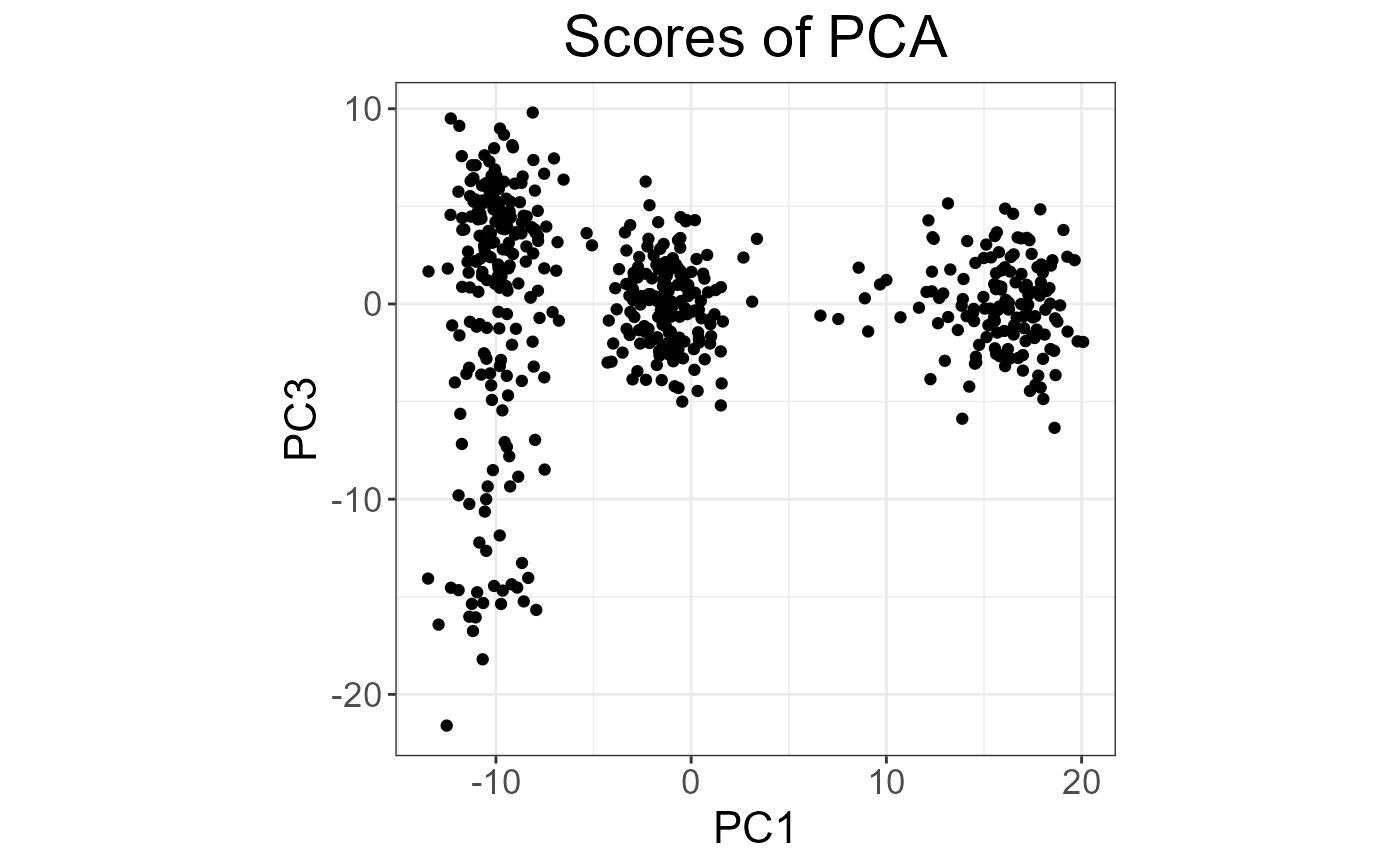plot(svd, type = "scores", scores = 1:4, ncol = 2, coeff = 0.7)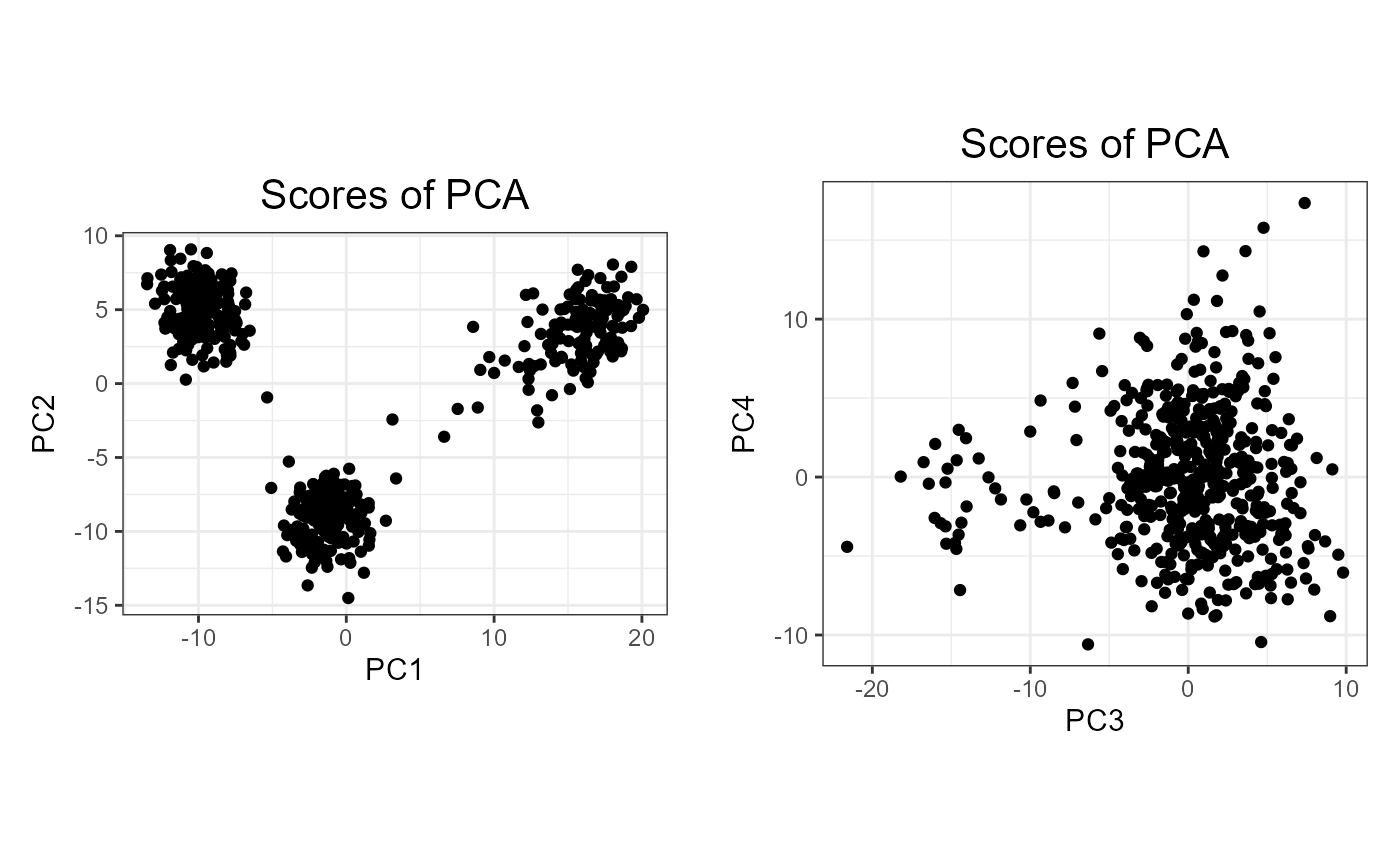## add color (recall that this return a ggplot2 object) class(obj <- plot(svd, type = "scores"))
#>  "gg" "ggplot"
pop <- rep(c("POP1", "POP2", "POP3"), c(143, 167, 207)) library(ggplot2) print(obj2 <- obj + aes(color = pop) + labs(color = "Population"))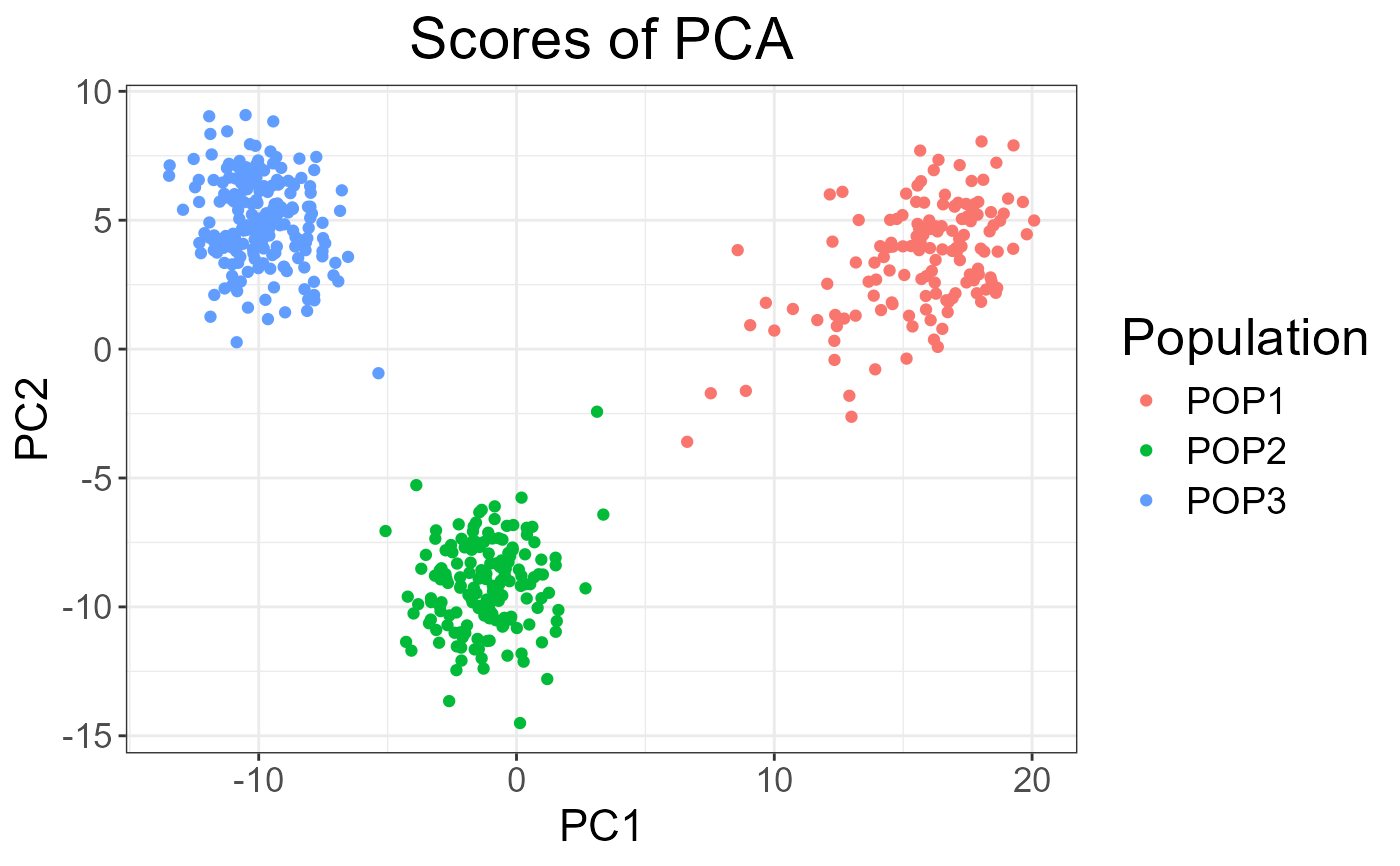## change the place of the legend print(obj3 <- obj2 + theme(legend.position = c(0.82, 0.17)))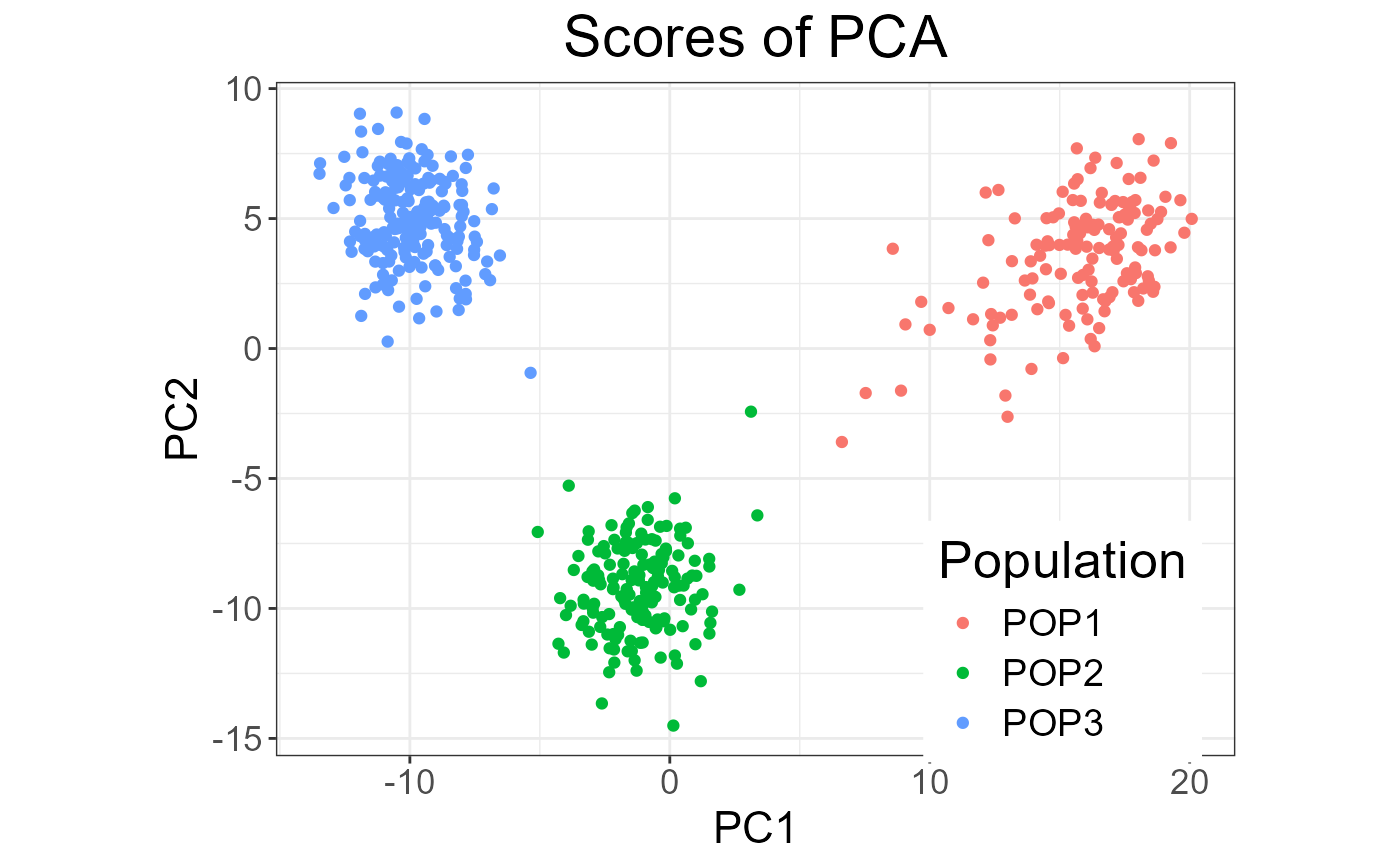## change the title and the labels of the axes obj3 + ggtitle("Yet another title") + xlab("with an other 'x' label")